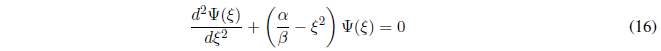We set up the Schödinger equation: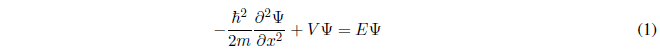The harmonic oscillator is subjected to a potential of the type: $V(x)=\frac{1}{2}kx^2$Solving for the derivative of higher degree, equation (2) can be written:We simplify equation (3) by defining the following magnitudes:Substituting (4) and (5) into (3):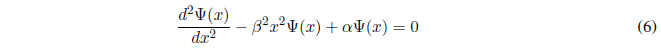Solving equation (6) requires the following change of variable: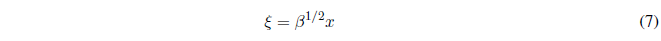Applying the chain rule:Solving for x from (7) and differentiating with respect to $\xi$ we obtain:Substituting (11) and (12) in (9) and solving for $\frac{d^2\Psi}{dx^2}$:Substituting (10) and (13) in (6):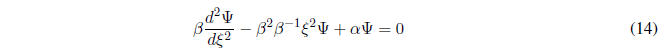Dividing (14) by $\beta$:By taking a common factor from the wave function, the Hermite-Gauss equation is obtained, known in Mathematics even before the birth of quantum mechanics.